### IMO Shortlist 2009 problem A1

Kvaliteta:
Avg: 0,0
Težina:
Avg: 6,0
Find the largest possible integer$k$, such that the following statement is true:
Let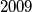$2009$ arbitrary non-degenerated triangles be given. In every triangle the three sides are coloured, such that one is blue, one is red and one is white. Now, for every colour separately, let us sort the lengths of the sides. We obtain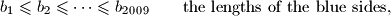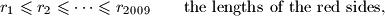Then there exist$k$ indices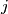$j$ such that we can form a non-degenerated triangle with side lengths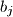$b_j$,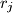$r_j$,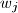$w_j$.

Proposed by Michal Rolinek, Czech Republic
Izvor: Međunarodna matematička olimpijada, shortlist 2009